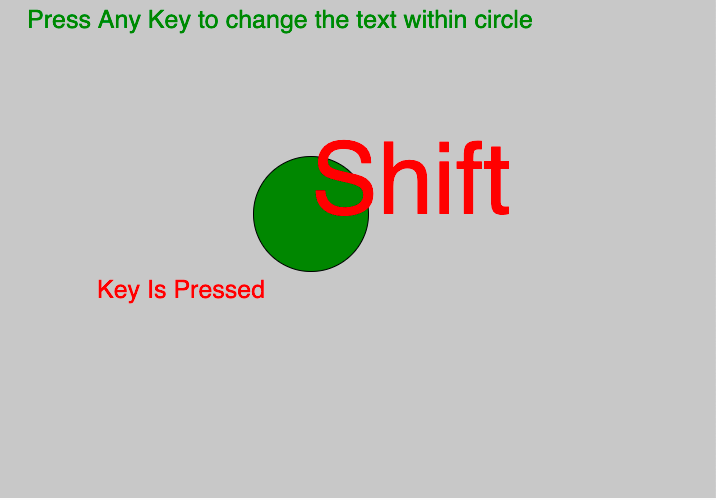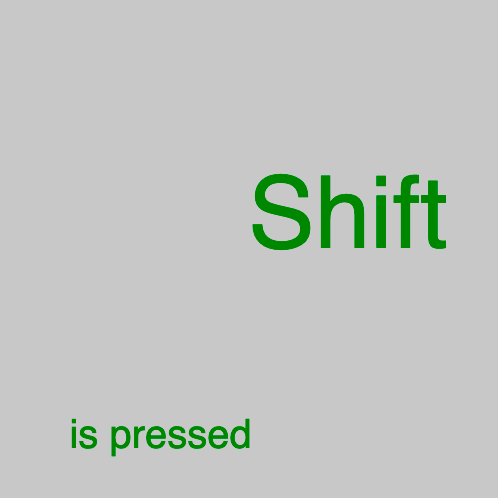Open In App
Related Articles
• Write an Interview Experience
• p5.js
• p5.js Introduction

# p5.js Keyboard key

The key variable in p5.js always contains the value of the key which is recently pressed. To get the proper capitalisation, it is best to use it within keyTyped(). Use the keyCode variable For non-ASCII keys.

Syntax:

```key
```

Below program illustrates the key variable in p5.js:
Example-1:

 `let valueX;``let valueY;`` ` `function` `setup() {``   ` `    ``// Create Canvas of size 500*500``    ``createCanvas(1000, 500);``}`` ` `function` `draw() {``   ` `    ``// set background color``    ``background(200);``   ` `    ``fill(``'green'``);``    ``// set text and text size``    ``textSize(25);``    ``text(``      ``'Press Any Key to change the text within circle'``      ``, 30, 30);``   ` `    ``// use of keyIsPressed Variable``    ``if` `(keyIsPressed) {``        ``// draw ellipse  ``        ``ellipse(mouseX, mouseY, 115, 115);``        ``fill(``'red'``);``        ``text(``"Key Is Pressed"``, 100, 300);``        ``textSize(100);``        ``text(key, mouseX, mouseY);``    ``} ``   ` `    ``else` `{``        ``rect(mouseX / 2, mouseY / 2, 300, 200);``        ``text(key, mouseX, mouseY);``    ``}`` ` `}`

Output:Example-2:

 `let valueX;``let valueY;`` ` `function` `setup() {``   ` `    ``// Create Canvas of size 500*500``    ``createCanvas(500, 500);``}`` ` `function` `draw() {``   ` `    ``// set background color``    ``background(200);``   ` `    ``fill(``'green'``);``   ` `    ``// set text and text size``    ``textSize(100);``   ` `    ``text(key, height / 2, width / 2);``    ``if` `(keyIsPressed) {``        ``textSize(40);``        ``text(``"is pressed"``, height / 7, width - 50);``    ``}`` ` `}`

Output:Reference: https://p5js.org/reference/#/p5/key